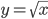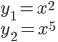### Day 76 - Arc Length and Surface Area - 12.10.14

• Unit 5/6 Test
• Average = 82%

Bell Ringer Quiz
• Volume
1. Set up and evaluate the integral that gives the volume of the solid formed by revolving the region about the x-axis.on the interval [1, 6]

1. 18

2. 17.5

3. 18π

4. 35π/2

5. none of the above

2. Set up and evaluate the integral that gives the volume of the solid formed by revolving the region about the x-axis bounded by.

1. 6/55

2. 0.10909...

3. 6π/55

4. π/5

5. none of the above

Review
• Prerequisites
• Integration
• Area Between Two Curves
• Volume
• Disk Method
• Washer Method

Lesson
• Arc Length
• Surface Area

Exit Ticket
• Posted on the board at the end of the block.
Lesson Objective(s)
• How can the arc length of a smooth curve and area of a surface revolution be calculated?

#### In-Class Help Requests

Standard(s)
• APC.9

• Apply formulas to find derivatives.

• Includes:

• derivatives of algebraic, trigonometric, exponential, logarithmic, and inverse trigonometric functions

• derivations of sums, products, quotients, inverses, and composites (chain rule) of elementary functions

• derivatives of implicitly defined functions

• higher order derivatives of algebraic, trigonometric, exponential, and logarithmic, functions

Math
ematical Practice(s)
• #1 - Make sense of problems and persevere in solving them
• #2 - Reason abstractly and quantitatively
• #5 - Use appropriate tools strategically
• #6 - Attend to precision
• #8 - Look for and express regularity in repeated reasoning

Past Checkpoints

Equations

Area Bounded by Two Functions

Disk Method

Washer Method